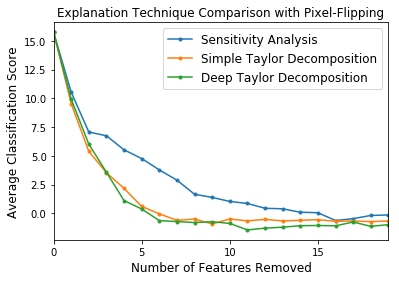/ Machine Learning

# Tensorflow tutorial for various Deep Neural Network visualization techniques## Understanding NN

This repository is intended to be a tutorial of various DNN interpretation and explanation techniques. Explanation of the theoretical background as well as step-by-step Tensorflow implementation for practical usage are both covered in the Jupyter Notebooks. I did not include explanation for techniques for which I thought the algorithm as well as the explanation of the original paper was clear.

UPDATE

It seems that Github is unable to render some of the equations in the notebooks. I strongly recommend using the nbviewer until I find out what the problem is (you can also download the repo and view them on your local environment). Links are listed below.

## 1 Activation Maximization

This section focuses on interpreting a concept learned by a deep neural network (DNN) through activation maximization.

### 1.1 Activation Maximization (AM)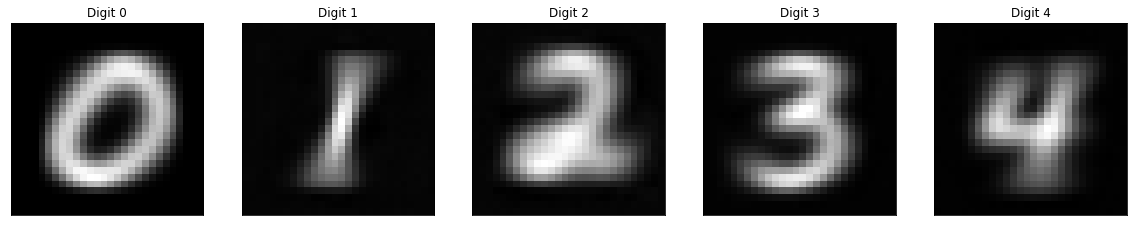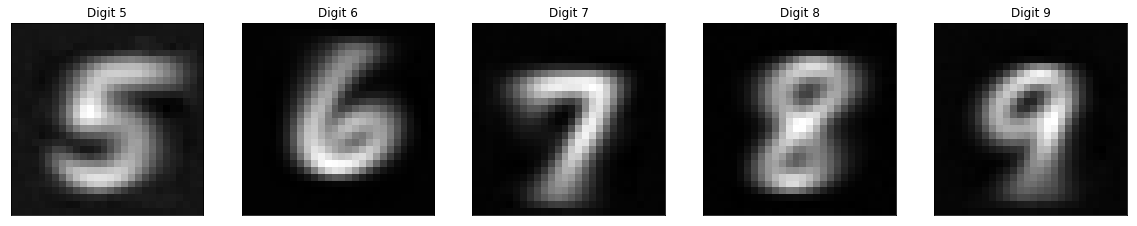### 1.3 Performing AM in Code Space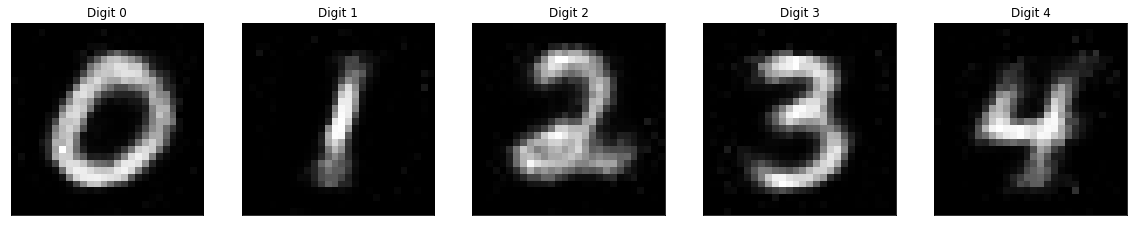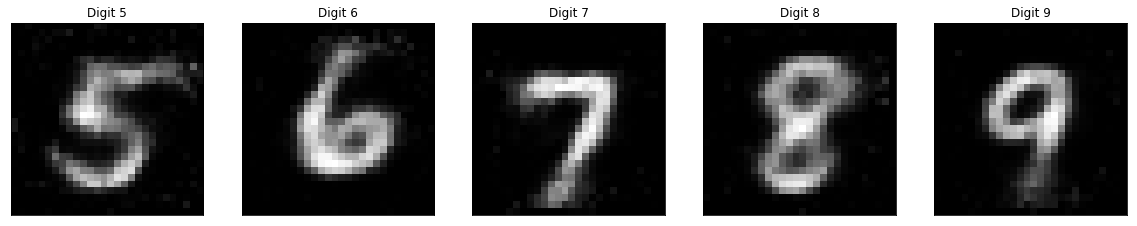## 2 Layer-wise Relevance Propagation

In this section, we first introduce the concept of relevance score with Sensitivity Analysis, explore basic relevance decomposition with Simple Taylor Decomposition and then build up to various Layer-wise Relevance Propagation methods such as Deep Taylor Decomposition and DeepLIFT.

### 2.1 Sensitivity Analysis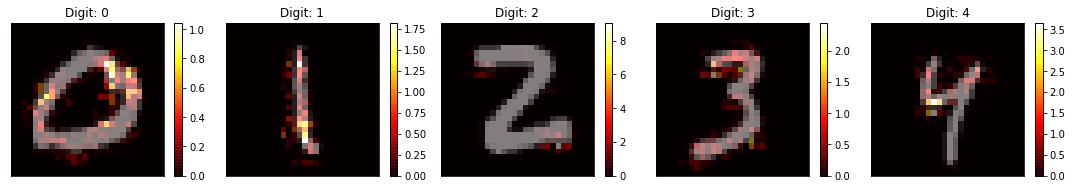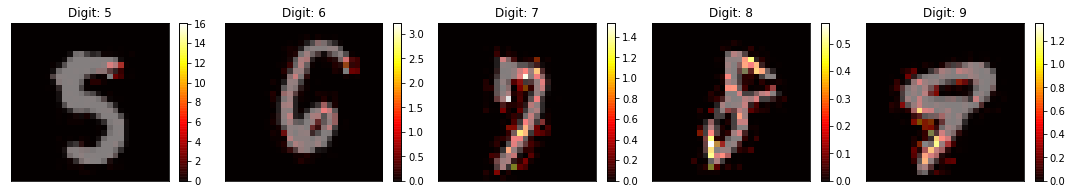### 2.2 Simple Taylor Decomposition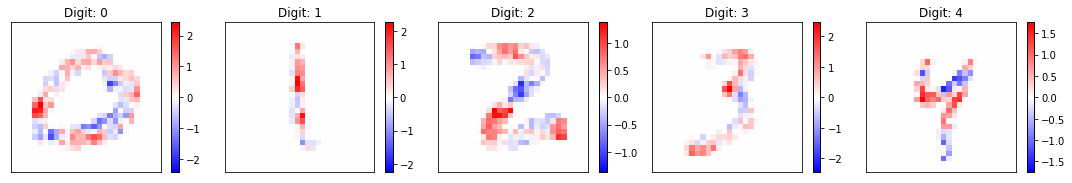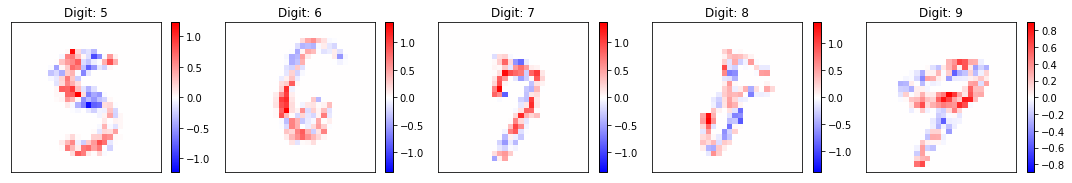### 2.3 Layer-wise Relevance Propagation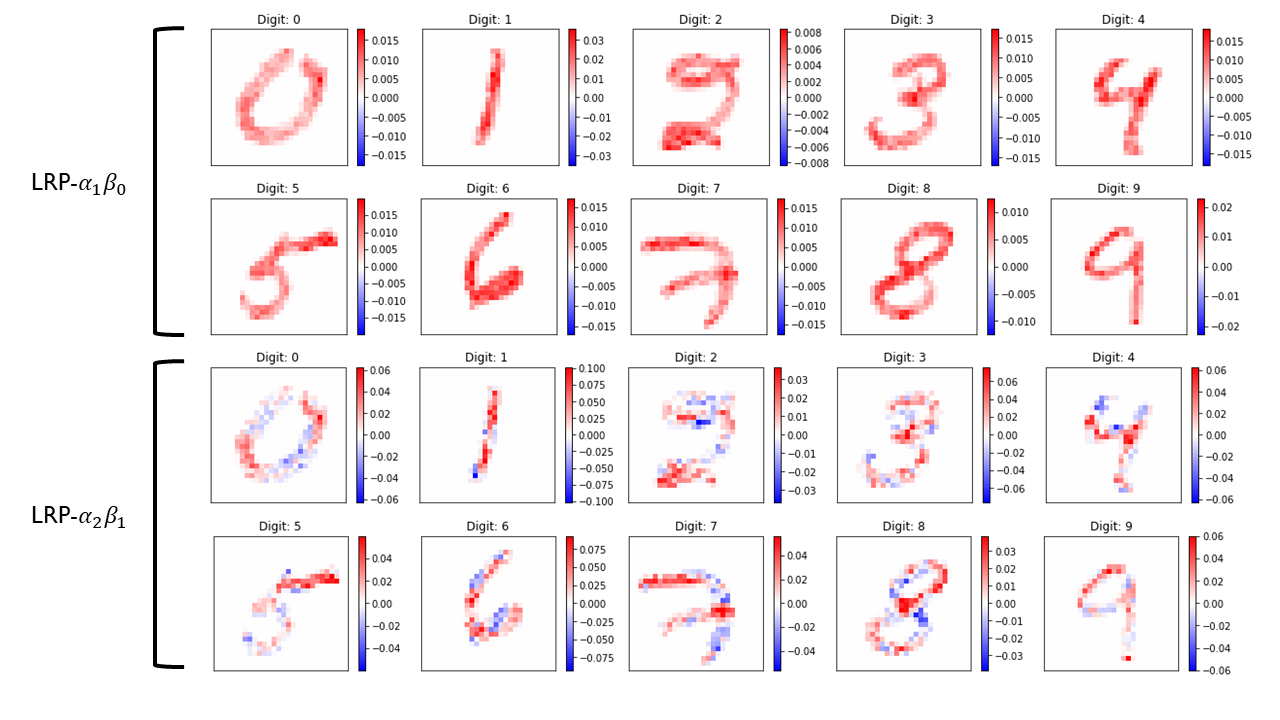### 2.4 Deep Taylor Decomposition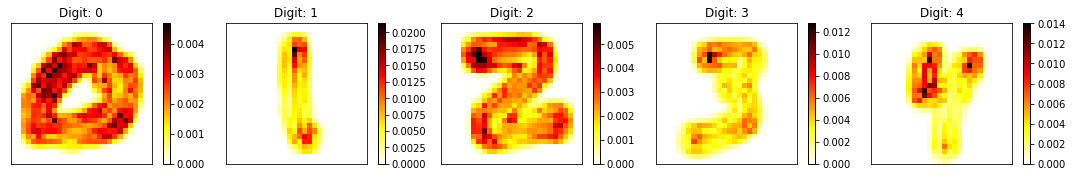### 2.5 DeepLIFTImplementation of various types of gradient-based visualization methods such as Deconvolution, Backpropagation, Guided Backpropagation, Integrated Gradients and SmoothGrad. Check out grad.py, a modular implementation of various gradient-based visualization techniques.

### 3.1 Deconvolution### 3.2 Backpropagation### 3.3 Guided Backpropagation## 4 Class Activation Map

Implementation of Class Activation Map (CAM) and its generalized versions, Grad-CAM and Grad-CAM++ the cluttered MNIST dataset.

### 4.1 Class Activation Map## 5 Quantifying Explanation Quality

While each explanation technique is based on its own intuition or mathematical principle, it is also important to de fine at a more abstract level what are the characteristics of a good explanation, and to be able to test for these characteristics quantitatively. We present in Sections 5.1 and 5.2 two important properties of an explanation, along with possible evaluation metrics.

### 5.1 Explanation Continuity### 5.2 Explanation Selectivity